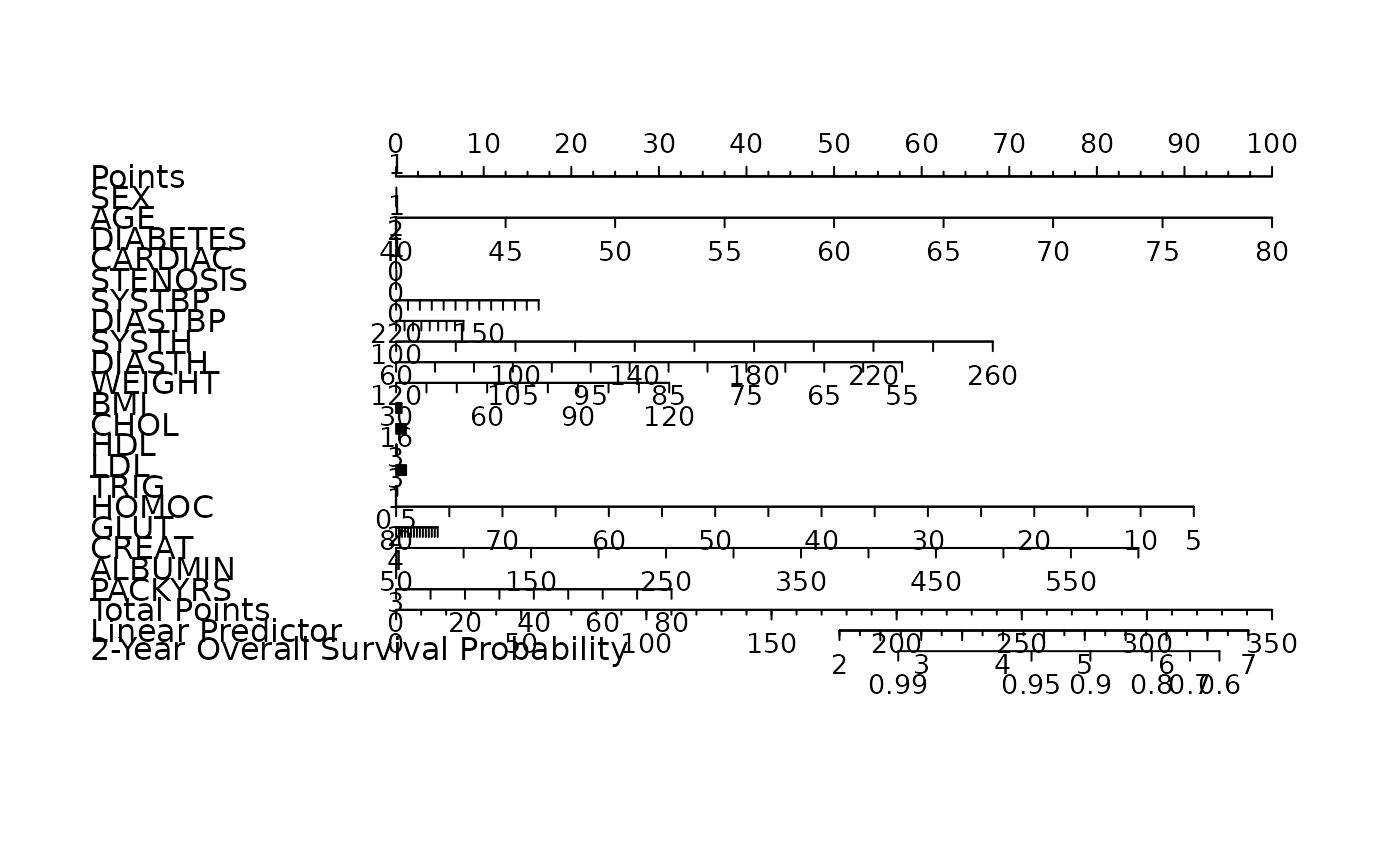Automatic model selection for high-dimensional Cox models with fused lasso penalty, evaluated by cross-validated likelihood.

fit_flasso(x, y, nfolds = 5L, lambda1 = c(0.001, 0.05, 0.5, 1, 5),
lambda2 = c(0.001, 0.01, 0.5), maxiter = 25, epsilon = 0.001,
seed = 1001, trace = FALSE, parallel = FALSE, ...)

## Arguments

x Data matrix. Response matrix made by Surv. Fold numbers of cross-validation. Vector of lambda1 candidates. Default is 0.001, 0.05, 0.5, 1, 5. Vector of lambda2 candidates. Default is 0.001, 0.01, 0.5. The maximum number of iterations allowed. Default is 25. The convergence criterion. Default is 1e-3. A random seed for cross-validation fold division. Output the cross-validation parameter tuning progress or not. Default is FALSE. Logical. Enable parallel parameter tuning or not, default is FALSE. To enable parallel tuning, load the doParallel package and run registerDoParallel() with the number of CPU cores before calling this function. other parameters to cvl and penalized.

## Note

The cross-validation procedure used in this function is the approximated cross-validation provided by the penalized package. Be careful dealing with the results since they might be more optimistic than a traditional CV procedure. This cross-validation method is more suitable for datasets with larger number of observations, and a higher number of cross-validation folds.

## Examples

data("smart")
x <- as.matrix(smart[, -c(1, 2)])[1:120, ]
time <- smart$TEVENT[1:120] event <- smart$EVENT[1:120]
y <- survival::Surv(time, event)

fit <- fit_flasso(
x, y,
lambda1 = c(1, 10), lambda2 = c(0.01),
nfolds = 3, seed = 11
)#> 123123
nom <- as_nomogram(
fit, x, time, event,
pred.at = 365 * 2,
funlabel = "2-Year Overall Survival Probability"
)

plot(nom)